# 22. Anharmonic Oscillators

Michael Fowler

## Introduction

Landau (para 28) considers a simple harmonic oscillator with added small potential energy terms. We'll simplify slightly by dropping the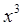term, to give an equation of motion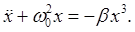(We'll always take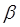positive, otherwise only small oscillations will be stable.)

We'll do perturbation theory (following Landau):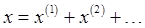(Standard practice in most books would be to write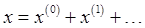with the superscript indicating the order of the perturbation -- we're following Landau's notation, hopefully reducing confusion...)

We take as the leading term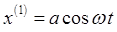with the exact value of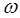,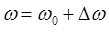. Of course, we don't know the value ofyet -- this is what we're trying to find. But even if we did somehow have the value ofexactly right, this expression would not be a full solution to the equation: the motion is certainly periodic with period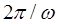, but the complete description of the motion is a Fourier series including frequenciesan integer, since the potential is no longer simple harmonic.

Putting this correct frequency into the equation gives a nonzero left hand side, so we rearrange. We subtract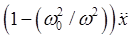from both sides to get: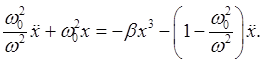Putting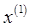into the left-hand side now gives zero: if the equation had zero on the right hand side, this would just be a free (undamped) oscillator with natural frequency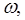notNow we can do perturbation theory. The equation for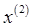is: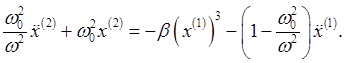Notice that the second term on the right hand side includes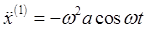. This equation now represents a driving force on an undamped oscillator exactly at its resonant frequency, so would cause the amplitude to increase linearly, obviously an unphysical result, since we're just modeling a particle sliding back and forth in a potential, no energy being supplied from outside.

The key is that there is also a resonant driver in that first term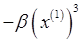. Clearly these two driving terms have to cancel, and this requirement nails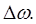Here's how: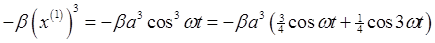so the resonant driving terms cancel provided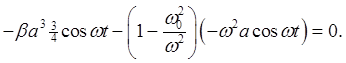Remembering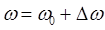, this gives (to this order)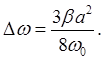(Actuallyin the denominator, but that's a higher order correction.)

Note that the frequency increases with amplitude: the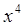potential gives an increasingly stronger restoring force with amplitude than the harmonic well.

## Resonance in a Damped Driven Oscillator: a Brief Review

The linear damped driven oscillator:(Following Landau's notation here -- note it means the actual frictional drag force is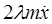.)

Looking near resonance for steady state solutions at the driving frequency, with amplitude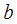, phase lag, that is,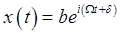, we find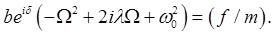For a near-resonant driving frequency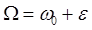and assuming the damping to be sufficiently small that we can drop the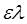term along with, the leading order terms give,

so the response, the dependence of amplitude of oscillation on frequency, is to this accuracy(We might also note that the resonant frequency is itself lowered by the damping, but this is another second-order effect we ignore here.)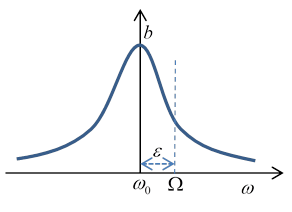The rate of absorption of energy equals the frictional loss. The friction forceon the mass moving at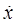is doing work at a rate:The half width of the resonance curve as a function of driving frequency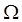is given by the damping. The total area under the curve is independent of damping.

For future use, we'll write the above equation for the amplitude in terms of deviation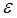from the resonant frequency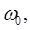## Damped Driven Nonlinear Oscillator: Qualitative Discussion

We'll take a damped, driven, nonlinear oscillator, one with a positive quartic potential term, as discussed above: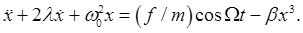First, a general discussion. We know from the results above that as the amplitude of oscillations increases, so does the frequency. As previously discussed, an increased oscillation amplitude encounters a potential becoming stronger and stronger than the simple harmonic oscillator.

So if we drive the oscillator from rest at the frequency that resonates with its small amplitude oscillations (where the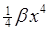potential term has negligible effect), as the amplitude builds up, the oscillator frequency increases, and the driving force falls out of sync.

The way to keep the amplitude increasing is evidently to gradually increase the frequency of the driving force to match the natural frequency at the increased amplitude. (Side note: this is the principle of the synchrocyclotron -- except, in that case the frequency is lowered as the energy increases, because the particles go to bigger and bigger orbits as their masses increase relativistically.) This way a small external driving force (enough to overcome frictional damping) can maintain a large amplitude oscillation at a frequency well above the frequency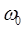of small oscillations.But what if we apply this high frequency to a system initially at rest, rather than gradually ramping up in sync with the oscillations? Then for a small driving force, we can treat the system as a damped simple harmonic oscillator, and this off-resonance force will drive relatively small amplitude oscillations.

The bottom line is that for the same external driving force, with frequency in some range above, there are two possible steady state oscillation amplitudes, depending on the history of the system.

Question: if it's the same driving force, how do you account for the fact that the large oscillation clearly does more work against friction?

Answer: the work done by the driving force depends on the phase difference between the force and the motion. It has to be different for the two cases.

## Nonlinear Case: Analysis

The equation of motion is:We established earlier that the nonlinear term brings in an amplitude-dependent correction to the oscillator's frequency: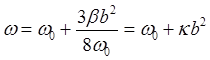in Landau's notation. (Actually thisis the same as our previous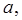but that's because we've slightly simplified Landau's treatment, we use just ananharmonicity term, he includes also anterm.)

The equation for the amplitude in the linear case (from the previous section) was, with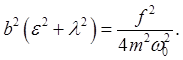For the nonlinear case, the maximum amplitude will clearly be at the true (amplitude dependent!) resonance frequency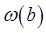so withas before, we now have a cubic equation for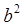: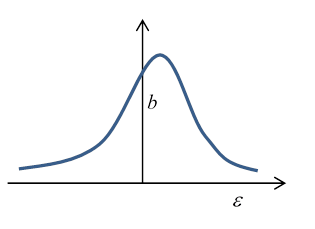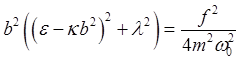.

Note that for small enough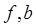is small (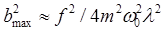) but the center of the peak has shifted slightly upwards, to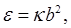that is, at a driving frequency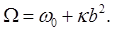The cubic equation forhas only this one real solution.

However, as the driving frequency is further increased, the coefficients of the cubic equation change and at a certain point two more real roots appear.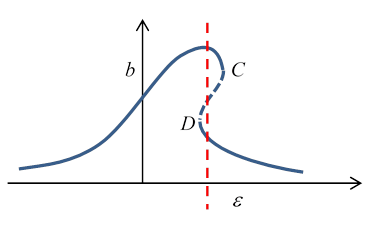The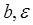curve for this larger driving force looks like:

So what's going on here? For a range of frequencies, including the vertical dashed red line in the figure, there appear to be three possible amplitudes of steady oscillation at one frequency. However, it turns out that the middle one is unstable, so will exponentially deviate, going to one of the other two, both of which are stable.

If the oscillator is being driven at its natural frequency, then the driving frequency is gradually increased, it will follow the upper curve to the point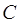, then drop discontinuously to the lower curve. Further frequency increase (with the same amplitude driving force, of course) will give diminishing amplitude of oscillation -- just as happens for the ordinary simple harmonic oscillator on going away from the resonant frequency.

If the frequency is now gradually lowered, the amplitude gradually will increase to point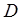, where it will jump discontinuously to the upper curve. The overall response to driving frequency is sometimes called hysteresis, by analogy with the response of a magnetic material to a varying imposed external field.

## Frequency Multiples

The above analysis is for frequencies not very far from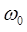. But nonlinear terms can cause resonance to occur at frequencies which are rational multiples of. Landau shows that a smallin the potential (so an additional force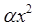in the equation of motion) can generate a resonance near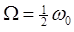. We've only considered a quartic addition to the potential,, a force, we can show that gives a resonance near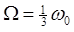.

We haveWe'll writeLet's define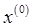by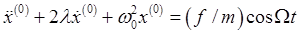So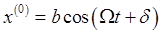. Then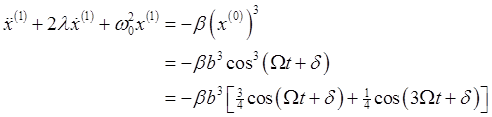Then, for, the second term,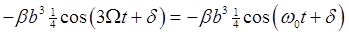, will have a resonant response, although it is proportional to the (small) amplitude cubed, so may not be dramatic.

Similar arguments work for other fractional frequencies.